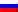# Time complexity

Contenus

## Time complexity

Time complexity asymptotically represents the time taken for an algorithm to find a solution.

Let a problem P and M be a method to solve this problem. The algorithm is a description with control and data structures making it possible to write the method M in a language recognizable by any individual or machine.

As a reminder, the control structures are: a sequence, a branch (or selection), a loop (or iteration). Data structures are: constants, variables, arrays (or ordered storage space), recursive structures (lists, graphs etc.).

## Elementary operation

The complexity consists in evaluating the effectiveness of the method M and comparing the latter with another method M '. This comparison is independent of the environment (machine, system, compiler, language, etc.). Efficiency depends on the number of elementary operations. These depend on the size of the data and the nature of the data.

Let n denote the size of the data and T (n) the number of elementary operations. The effectiveness evaluation will be done in the best case, the worst case and the average case.

In the case of a sequence type structure, the evaluation is equal to the sum of the costs. For example, if the algorithm has processing T1 (n) followed by T2 (n), then T (n) = T1 (n) + T2 (n).

In the case of a branch, the evaluation is equal to the maximum of branches. For example, the algorithm executes T1 (n) otherwise T2 (n), then T (n) = max (T1 (n), T2 (n)).

In the case of a loop, the evaluation is equal to the sum of the costs of the successive passages. For example, if the algorithm is a "while doing Ti (n) with the complexity of Ti (n) depending on the number i of the iteration. Then the complexity is T (n) = sum (i = 1 to n) Ti (n).

For a recursive version, I invite you to go to the corresponding page. Let C (n) be the processing performed in a divide & conquer function. Then the complexity is T (n) = 2 * T (n / 2) + C (n) = n log (n) if C (n) = n.

## Landau notation

Landau's notation characterizes the asymptotic behavior of a function, ie the behavior of f (n) when n tends to infinity.

We say that f (n) is a large O of g (n) if.

## Worse, Better and Average

We can calculate for most algorithms a complexity in the worst case (the greatest number of elementary operations), the best and on average. The mean is a sum weighted by the probability of the presence of possible complexities. Most often, we use the worst case complexity because we want to know an upper limit of execution time.

Consider an algorithm for finding an element in an array in iterative form. The algorithm stops when the value has been found. The algorithm breaks down as follows: assignment of 0 to i, as long as i has not traversed the array we increment i, if tab [i] = value then we return true, otherwise at the end of the array we return false. Let C denote the complexity of the body of the loop.

In the worst case, the algorithm traverses the whole table, that is to say that T (n) = 1 + n * C = O (n). At best, the element is at the start of the array, so T (n) = 1 + C = O (1). We consider that on average, there is a 50% chance that the element is not in the array, and 50% that it is in the middle of the array (this is of course absurd, but we will not do all the possibilities ). In this case, the complexity on average is T (n) = 0.5 * (1 + n * C) + 0.5 * (1 + n * C / 2) = a * n + b with a and b constants = O (not). We notice that the asymptotic behavior on average is the same as in the worst case.

## Examples

Suppose that each of the expressions below gives the processing time T (n) spent by an algorithm to solve a problem of size n. Select the dominant term (s) with the greatest increase in n and specify the lowest Big-Oh complexity of each algorithm.

The instructions below show some characteristics of the “Big-Oh” notation for the functions f ≡ f (n) and g ≡ g (n). Determine if each statement is TRUE or FALSE and correct the formula in the latter case.FRFRENRUES